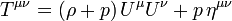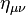## Saturday, April 21, 2012

### Lagrangian WorldsDiagram of the Lagrange Point gravitational forces associated with the Sun-Earth system.

In a certain sense a perfect fluid is a generalization of a point particle. This leads to the question as to what is the corresponding generalization for extended objects. Here the lagrangian formulation of a perfect fluid is much generalized by replacing the product of the co-moving vector which is a first fundamental form by higher dimensional first fundamental forms; this has as a particular example a fluid which is a classical generalization of a membrane; however there is as yet no indication of any relationship between their quantum theories.A Fluid Generalization of Membranes.

# Perfect fluid

In physics, a perfect fluid is a fluid that can be completely characterized by its rest frame energy density ρ and isotropic pressure p.
Real fluids are "sticky" and contain (and conduct) heat. Perfect fluids are idealized models in which these possibilities are neglected. Specifically, perfect fluids have no shear stresses, viscosity, or heat conduction.
In tensor notation, the energy-momentum tensor of a perfect fluid can be written in the form$T^{\mu\nu} = (\rho + p) \, U^\mu U^\nu + p \, \eta^{\mu\nu}\,$
where U is the velocity vector field of the fluid and where$\eta_{\mu \nu}$ is the metric tensor of Minkowski spacetime.
Perfect fluids admit a Lagrangian formulation, which allows the techniques used in field theory to be applied to fluids. In particular, this enables us to quantize perfect fluid models. This Lagrangian formulation can be generalized, but unfortunately, heat conduction and anisotropic stresses cannot be treated in these generalized formulations.

Perfect fluids are often used in general relativity to model idealized distributions of matter, such as in the interior of a star.